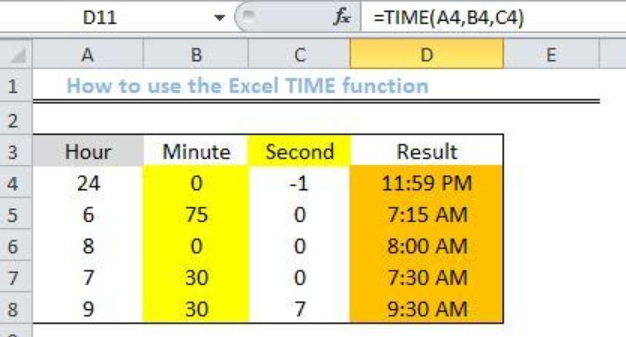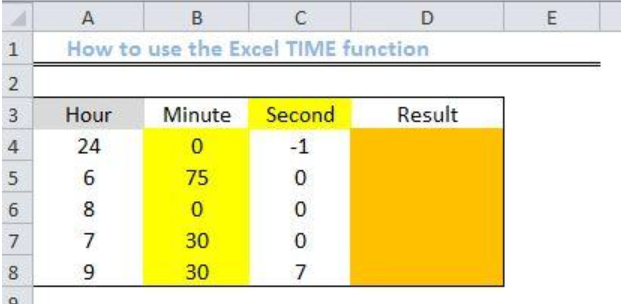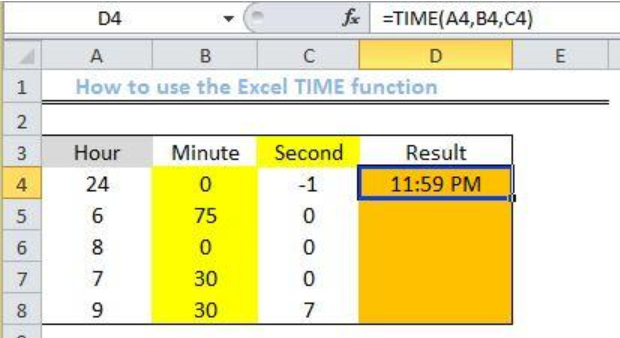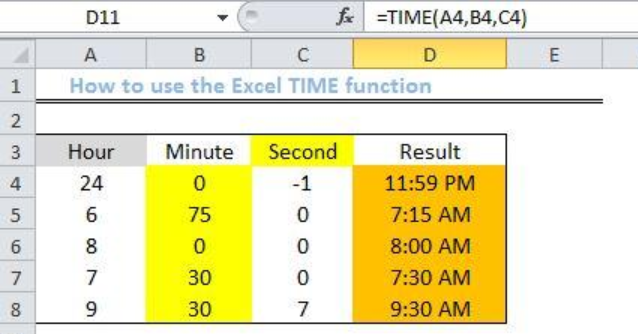Get instant live expert help with Excel or Google Sheets“My Excelchat expert helped me in less than 20 minutes, saving me what would have been 5 hours of work!”

#### Post your problem and you'll get expert help in seconds

Your message must be at least 40 characters
Our professional experts are available now. Your privacy is guaranteed.

# Excel TIME Function

We can use the Excel TIME function to create time using its individual components like hour, minutes and seconds values to return with a decimal number representing the specific time. The steps below will walk us through the process of using this in-built function.Figure 1- Result of using the Excel TIME function

## General Formula

`=TIME(hour,min,sec)`

## Formula

`=TIME(A4,B4,C4)`

## Setting up the Data

• We will create out a possible list of data as displayed in the figure below.
• Column A will contain a list of Hours
• Our list of Minutes will be displayed in Column B
• Column C will contain a list of Seconds
• We will insert our formula in Column D to arrive at the decimal result.Figure 2 – Setting up the Data

## Applying the Excel TIME function

• We will click on Cell D4
• We will insert the formula below into Cell D4
`=TIME(A4,B4,C4)`
• We press the enter keyFigure 3 – Applying the Excel TIME function

• Using the fill handle tool (a plus sign located at the base of Cell D4), we will drag down the formula into the other Cells.Figure 4 – Result of using the Excel TIME function

## Explanation

We use the TIME function to create a serial number format (decimal value) from its hours, minutes and seconds component. However, you must always use a valid component, because a valid component will always return with a valid time which can be formatted in your own way.

Also, we can combine the TIME function with many other functions to perform different operations such as:

• Add decimal hours or decimal minutes to time through the use of the TIME function by adding the minutes or hours divided by 24 times
• Use the TIME function together with the SUMPRODUCT function to achieve the sum of the total amount of time over 30 minutes.
• Count the number of time in a given array by combining the TIME function and the COUNTIFs function.

## Note

• Negative values are OK
• When minutes or seconds are over 60, they will be rolled over to the hour or minute respectively.
• When there are no components of some cells in a particular row, then zero will be inserted in the empty cells to prevent an error result.
• The returned time will be in decimal form. Right-click and click on format cells. Click on time and select the time format.

## Instant Connection to an Expert through our Excelchat Service

Most of the time, the problem you will need to solve will be more complex than a simple application of a formula or function. If you want to save hours of research and frustration, try our live Excelchat service! Our Excel Experts are available 24/7 to answer any Excel question you may have. We guarantee a connection within 30 seconds and a customized solution within 20 minutes.

### Did this post not answer your question? Get a solution from connecting with the expert.Another blog reader asked this question today on Excelchat:## Subscribe to Excelchat.coAnother blog reader asked this question today on Excelchat: